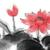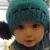## js里的原型继承问题

为什么80%的码农都做不了架构师？->>>function A(x){
this.x=x;
}

A.prototype.getx=function(){
return this.x;
}

function B(x,y){
this.y=y;
A.call(this,x);
}

B.prototype=new A();/*疑问*/
var b=new B(1,2);

B.prototype=A.prototype;

1```var a = {
}```

`var b = a;`

`a.prototype = b.prototype;`

1B.prototype=A.prototype;

0
C